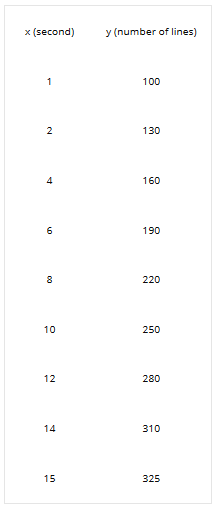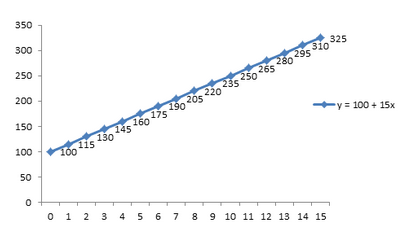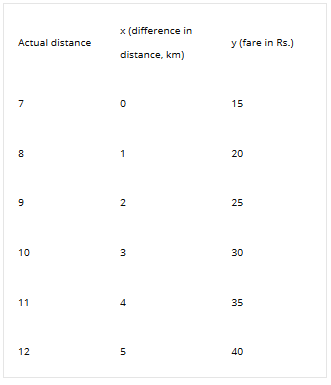Courses

# Representation of Functions Mathematics Notes | EduRev

## Algebra for IIT JAM Mathematics

Created by: Veda Institute

## Mathematics : Representation of Functions Mathematics Notes | EduRev

The document Representation of Functions Mathematics Notes | EduRev is a part of the Mathematics Course Algebra for IIT JAM Mathematics.
All you need of Mathematics at this link: Mathematics

Representation of Functions
The function is the connection or the link between two sets and can be represented in different ways. Consider the above example of the printing machine. The function that shows the relationship between the numbers of seconds (x) and the numbers of lines printed (y). We are quite familiar with functions and now we will learn how to represent them.

Algebraic Representation
Here, we will represent the function by a simple algebraic equation which is: f(x) = y = 100 + 15(x). For different values of x, the values of y (= f(x)) change accordingly. What if, one wants to know about the numbers of lines printed by the machine in 15 seconds? Simple, Numbers of words printed = y = 100 + 15 (15) = 325.

Table Representation
In this method, we represent the relationship in the form of a table. For each value of x (input), there is one and only one value of y (output). The table representation of the problem:Graphical Representation
Here, we will draw a graph showing the connection between the two elements of two sets say x and y such that x ∈ X and y ∈ Y. Plotting the satisfying points of x and y in the respective axes. Drawing a straight line passing through these points will represent the function in a graphical way. Graphical representation of the above problem:Solved Examples for You
Problem: Consider an auto-driver who charges Rs. 15 for the first 7 km and subsequently charges an additional fare of Rs. 5 for each km. Find the cost one has to pay for 12 km by representing in tabular form.
Solution: Let x be the difference in distance (km) the auto ran and y be the fare of the auto for different distances (in Rs.). The equation of the given problem is: f(x) = y = 15 + 5 (x). The difference in distance travelled by auto is 12 – 7 = 5 km and we are required to find the fare for it. In tabular form, the above problem isOne has to pay Rs. 40 for travelling a distance of 12 km.

Offer running on EduRev: Apply code STAYHOME200 to get INR 200 off on our premium plan EduRev Infinity!

,

,

,

,

,

,

,

,

,

,

,

,

,

,

,

,

,

,

,

,

,

;